# CDS 2013 Maths Question Paper -1

1. Consider the following 1 statements :
2. There is A finite number of rational number? Between any two rational numbers.
3. There is an infinite number of rational numbers between any two rational numbers.
4. There is a finite number of irrational numbers between any two rational numbers.

Which of the above statements is /are correct ?

• I only
• 2 only
• 3 only
• 2 and 3
1. Representation of 0.2341 in the form p/q where p, q are integers, q ≠ 0, is
• 781/3330
• 1171/4995
• 2341/9990
• 2339/9990
1. In a school there are 30 teachers who teach Mathematics or Physics. Of these teachers. 20 teach Mathematics and 15 teach Physics, 5 teach both Mathematics and Physics, The number of teachers teaching only Mathematics is
• 5
• 10
• 15
• 20
1. ‘X’ can complete a job in 12 days. If ‘X’ and Y work together, they can complete the job in 6 2/3 days. ‘y’ alone can complete the job in
• 19 days
• 12 days
• 15 days
• 18 days
1. If a body covers a distance at the rate of x km/hr and another equal distance at the rate of y km/hr, then the average speed in km/hr is.
• X+y/2
• √xy
• 2xy/x+y
• X+y/xy
1. A sailor sails a distance of 48 km along the flow of a river in 8 hours. If it takes 12 hours to return the same distance, then, the speed of the flow of the river is
• 5 km/hr
• 1 km/hr
• 5 km/hr
• 2 km/hr
1. A mason can build a tank in 12 hours. After working for 6 hours, he took the help of a boy and finished the work in another 5 hours, The time that the boy will take alone to complete the work is.
• 30 hrs
• 45 hrs
• 60 hrs
• 64 hrs
1. The principal on which a simple interest of Rs. 55 will be obtained after 9 months at the rate of 3 2/3% per annum is
• 1,000
• 1,500
• 2,000
• 2,500
2. The expression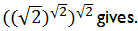• a natural number
• an integer and not a natural number
• a rational number but not an integer
• a real number hut not a rational Number
3. The I,CM of two numbers is 2376 while their HCF is 33. If one of the numbers is 297, then the other number is.
• 216
• 264
• 642
• 792
1. A person travels a certain distance at 3 km/hr and reaches 15 minutes late. If he travels at, 4 km/hr, he reaches 15 minutes earlier. The distance he has to travel is.
• 5 km
• 6 km
• 2 km
• 12 km
1. The two digit number, which when divided by sum of the digits and product of the digits respectively, the remainder is same, and the difference of quotients is one, is
• 14
• 23
• 32
• 41
1. The short and long hands of a dock are 4 cm and 6 cm long respectively. Then the ratio of distances travelled by tips of short hand in 2 days and long hand in 3 days is
• 4 : 9
• 2 : 9
• 2 : 3
• 1 : 27
1. What is tire value of 725x725x725+371x371x371/725×725-725×371+371×371 ?
• 9610
• 1960
• 1096
• 1016
1. If N, (N + 2), (N + 4) are prime numbers, then the number of possible solutions for N are
• 1
• 2
• 3
• None of the above
2. The sum of two numbers is 7 and the sum of their squares is 25. The product of the two numbers is
• 6
• 10
• 12
• 15
1. Tine system of equations 3x + y – 4 = 0 and 6x+2y-8=0 has
• a unique solution x -1,y = 1
• a unique solution x = 0, y = 4
• no solution
• infinite solutions
1. (a+1)4 – a4 is divisible by
• -2a2 + 2a – 1
• 2a3 – 2a – 1
• 2a3 – 2a + 1
• 2a2 + 2a + 1
1. The, factors (s) of 5px – l0qy + 2rpx – 4qry is/are
• (5+2r)only
• (px – 2qy) only
• Both (5 + 2r) and (px – 2qy)
• Neither (5 + 2r) nor (px – 2qy)
1. If one of the root* of the equation x2-bx+ c = 0 is the square of the other, then which one of the following is correct ?
• b3 = 3bc + c2 + c
• c3 = 3bc + b2 + b
• 3bc = c3 + b2 + b
• 3bc = c3 + b3 + b2

1. If x + 1/x = 2, then what is x – 1/x equal to ?
• 0
• 1
• 2
• – 2
1. What is the value of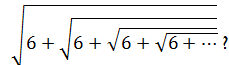?
• 2
• 3
• 5
• 4
2. One of the factors of the polynomial x4 – 7x3 + 5x2 – 6x + 81 is .
• x + 2
• x – 2
• x + 3
• x – 3
1. Let A= (x : x is an odd integer) and B = [x : x2 – 8x + 15 = 0). Then which one of the following is correct?
• A = B
• A ⊆ B
• B ⊆ A
• A ⊆ B0
2. Consider the following- in respect of the sets A and B .
3. (A ∩ B) ⊆ A
4. (A ∩ B) ⊆ B
5. A ⊆ (A ∪ B)

Which of the above are correct ?

• 1 and 2 only
• 3 and 3 only
• 1 and 3 only
• 1, 2 and 3
1. What is the HCF of the polynomials: x3+8, x2+5x+ 6 and x3+ 2x2 + 4x + 8 ?
• X+2
• X+3
• (x + 2)2
• None of the above
1. The set of natural numbers is closed under
3. subtraction
4. multiplication
5. division

Which of the above is/are correct ?

• 1 only
• 1 and 3 only
• 1,2 and 3
• 3 and 4 only
1. For what value of k, is (x + 5) a factor of 6x2 + kx + 10 ?
• 5
• 32
• 36
• 40
1. If 3 men and 4 boys can do a piece of work in 8 days, then 6 men 8 boys can do the same work, in
• 2 days
• 4 days
• 6 days
• 16 days
1. Y and Z had taken a dinner together. The cost of the meal of Z was 20% more than that of Y and the cost of the meal of X was 5/6 as much as the cost of the meal of Z. If X paid Rs.100, then what was the total amount that all the three of them had paid ?
• 285
• 300
• 355
• None of the above
2. Which one of the following numbers is divisible by 11?
• 45678940
• 54857266
• 87524398
• 93455120
3. Ten chairs and six tables together cost Rs.6,200 , three chairs and two tables together coat Rs.1,900. The cost of 4 chairs and 5 tables is.
• 3,000
• 3,300
• 3,500
• 3,800
1. If a, b, c, d, e are in continued proportion, then a/e is equal to
• a3/b3
• a4/b4
• b3/a3
• b4/a4

2. If the expression x3 + 3x2 +4x + p contains (x + 6) as a factor, then the value of p is.
• 132
• 141
• 144
• 151
1. The difference in the roots of the equation 2x2-11x+ 5 =0 is
• 5
• 4
• 5
• 3
1. The HOP of (x4 -y4)and ( x6-y6) is
• x2 – y2
• x-y
• x3 – y3
• x4 – y4

2. The LCM of (x3 – x2 – 2x) and (x3 +x2) is
• x3 – x2 – 2x
• x 2+ x
• x4 – x3 – 2x2
• x-2
1. The smallest positive prime (say p) such that 2P – 1 is not a prime is
• 5
• 11
• 17
• 29
2. A number consists of two digits whose sum is 10, If the digits of the number are reversed, then the number decreases by 36 Which of the following is/are correct ?
3. The number is divisible by a composite number.
4. The number is a multiple of a prime number.

Select the correct answer using the code given below :

• I only
• 2 only
• Both 1 and 2
• Neither 1 nor 2
1. if 16x 8n+2 – 2m, then m equals
• n + 8
• 2n+10
• 3n + 2
• 3n-10
2. Consider the following statements for 0 ≤ θ ≤  90°;
3. The value of sinθ + cos θ is always greater than 1.
4. The value of tanθ + cot θ is always greater than 1.

Which, of the above statements is/are correct ?

• 1 only
• 2 only
• Both 1 and 2
• Neither 1 nor 2
1. What is tan A-sin A/sin3 A equal to ?
• sec A/1 – cos A
• sac A/1+ cos2 A
• sec A/1 + cos A
• None of the above
1. If ain A = 3/5 and A is an acute angle, then tan A + sec A is equal to
• 0
• 1
• 2
• -1
1. If sin θ = x2-y2/x2+y2,then which one of the following is correct ?
• cosθ = 2xy/x2-y2
• cosθ = 2xy/x2+y2
• cosθ = x-y/x2+y2
• cosθ = xy(x-y)/x2+y2

1. if a2 = 1+2 sinθ cosθ  /1-2 sinθ  cos θ ,then what is a+1/a-1equal to?
• secθ
• 1
• 0
• tanθ
1. If cos A + cos2 A = 1, then what is 2(sin2 A + sin4 A) equal to ?
• 4
• 2
• 1
• ½
1. What is (1 – tan A)2 + (1 + tan A)2 + (1- cot A)2 +(l + cot A)2 equal to ?
• sin2 A cos2 A
• sec2 A cosec2 A
• 2 sec2 A cosec2 A
• None of the above

Direction: Consider the following far the, next four (04) questions that follow :

As seen from the top and bottom of a building of height h metres, the angles of elevation of top of a tower of height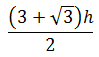metres are α and β respectively.

1. If β = 30° , then what is tan α equal to ?
• l/2
• 1/3
• ¼
• None of the above
1. If α = 30° , then what is tan β equal to ?
• 1
• 1/2
• 1/3
• None of the above
1. If α = 30° and h =30 m, then what is the distance between the base of the building and the base of the tower ?
• 15+15 √3 m
• 30+15√3 m
• 45+15√3 m
• none of the above
1. If  β = 30°and if θ is the angle of depression of the foot of the tower as seen from the top of the building, then what is tan θ equal to ?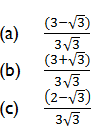• None of the above

Directions: Consider the following for the nest four (04) questions that follow :

The angles A, Bf C, D of a quadrilateral ABCD are in the ratio 1 : 2 : 4 : 5.

1. What is cos (A + B) equal to ?
• 0
• ½
• 1
• None of the above
2. What is cosec (C – D+ B) equal to ?
• 1
• 2
• 3
• 4
3. Consider the following statements :
4. ABCD is a cyclic quadrilateral. .
5. sin (B – A) = cos (D – C).

Which of the above statements is/are correct ?

• 1 only
• 2 only
• Both 1 and 2
• Neither 1 nor 2
1. What is sec2 D -tan2 D equal to ?
• ½
• 2/3
• 1
• None of the above
2. An isosceles right-angled triangle has area 1 square unit. What is its perimeter ?
• 3 units
• 2 √2 + 1 units
• √2 + 1 units
• 2 ( √2 + 1) units
3. If the heights and the areas of the base of a right circular cone and a pyramid with square base arc the same, then they have
• same volume and same surface area
• same surface area but different volumes
• same volume but different surface areas
• different volumes and different surface areas
4. A circular water fountain 6.6 m in diameter is surrounded by a path of width 1.5 m. The area of this path in square metres is
• 62π
• 15π
• 15π
• None of the above
5. The area of a rectangular field is 4500 square metres. If its length and breadth are in the ratio 9 : 5, then its perimeter is
• 90 m
• 150 m
• 280 m
• 360 m
6. The area of a square inscribed in a circle of radius 8 cm is
• 32 square cm
• 64 square cm
• 128 square cm
• 256 square cm
7. A solid spherical ball of iron of radius 4 cm melted to form spheres of radius 2 cm each The number of spheres so formed is
• 8
• 9
• 10
• 16
1. From a solid wooden right circular cylinder, a right circular cone whose radius and height are same as the radius and height of the cylinder respectively, is carved out. What is the ratio of the volume of the utilized wood to that, of the wasted wood ?
• 1 : 2
• 2: 1
• 2 : 3
• 1 : 3
2. The are AB of the circle with centre at ‘O’ and radius 10 cm has length 16 cm. What is the area of the sector bounded by the radii OA, OB and the arc AB ?
• 40π square cm
• 40π square cm
• 80π square cm
• 20π square cm
1. The height of a cylinder is 15 cm. The lateral surface area is 660 square cm. Its volume is
• 1155 cubic cm
• 1215 cubic cm
• 1230 cubic cm
• 2310 cubic cm
1. A bucket is of height 25 cm. Its top and bottom radii are 20 cm and 10 cm respectively. Its capacity in litres is
• 5 π/3
• 20π
• 25π
2. The volume of a right circular cone of height 3 cm and slant height 5 cm is
• 3 cubic cm
• 3 cubic cm
• 52 cubic cm
• 53 cubic cm
3. A cylindrical tube open at both ends is made of metal. The internal diameter of the tube is cm and length of the tube is 10 cm. If the thickness of the metal used is 1 cm, then the outer curved surface area of the tube is
• 140π square cm
• 5π square cm
• 70π square cm
• None of the above
4. The ratio of surface area to diameter of a sphere whose volume is 36π cubic cm is
• None of the above
5. The volume of the material of a hemispherical shell with outer and inner radii 9 cm arid cm respectively is approximately
• 808 cubic cm
• 800 cubic cm
• 816 cubic cm
• 824 cubic cm
6. The length, breadth and height, of a box are respectively 14 m, 12 m and 13 m- The length of the greatest rod that can be put in it is
• 31 m
• 56 m
• 20 m
• 5 m
1. Each side of an equilateral triangle is 6 cm its altitude is ?
• 6√3 cm
• 3√3 cm
• 2√3 cm
• √3 cm
1. What is the quantity of cloth required to roll up to form a right circular tent whose base is of radius 12 m and height 5m?
• 40π square metre
• 60π square metres
• 78π square metres
• 156π square metres
2. The‘minute hand of a watch is 2.5 cm long. The distance its extreme end traverses in 40 minutes is
• 10 π/3 cm
• 3 π/l0 cm
• 10/3 cm.
• 10 cm
3. A swimming pool 70 m long, 44 m wide and 3 m deep is filled, by water flowing from a pipe at the rate of 30,800 cm3 per second. The time taken to fill the swimming pool is
• 7l/2 hours
• 80 hours
• 250/3 hours
• None of the above
1. If the area of a regular hexagon 96√3  square cm, then its perimeter is
• 36 cm
• 48 cm
• 54 cm
• 64 cm
1. If the arms of one angle are respectively parallel to the arms of another angle, then the two angles are
• neither equal nor supplementary
• not equal but supplementary
• equal but not supplementary
• either equal or supplementary
1. The side BC of a triangle ABC is produced to D; bisectors of the angles ABC and ACD meet at P. If ∠BPC = x° and ∠BAC = y°, then which one of the following is correct ?
• x° – y°
• x° + y° = 90°
• x° + y° =180°
• 2x° = y°
1. There are 45 male and 15 female employees in an office. If the mean salary of the 60 employees is Rs.4,800 and the mean salary of the male employees is Rs.5,000, then, the mean salary of the female employees is
• 4,200
• 4,500
• 5,600
• 6,000
2. Pipe A can fill a tank in 10 minutes and pipe B can empty it in 15 minute. If both the pipes are opened m an empty tank, the time taken to make it full is
• 20 minutes
• 25 minutes
• 30 minutes
• None of the above
1. ABCD is a trapezium with, parallel sides AB = 2 cm and DC = 3 cm. E and F are the mid-points of the non-parallel aides. The ratio of area of ABFE to area of EFCD is
• 9 : 10
• 8 : 9
• 9 : II
• 11 : 9
1. In the -figure given above, PQRS is a parallelogram. If AP. AQ, OR, CS are bisectors of ∠P, ∠Q, ∠R and ∠S respectively, then ABCD is a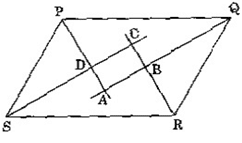• square
• rhombus
• rectangle
• None of the above
2. In the figure given above, the area of rectangle ABCD is 100 square cm, 0 is any point on AB and CD = 20 cm. Then the area of triangle COD is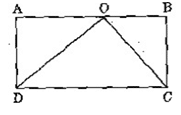• 40 square cm
• 45 square cm
• 50 square cm
• 80 square cm
1. ABC is a right-angled triangle such that AB =a – b, BC = a and CA = a + b. D is a point on BC such that BD = AB. The ratio of BD : DC for any value of a and b is given by
• 3:2
• 4:3
• 5 : 4
• 3:1
1. Let ABC be an equilateral triangle. If the side BC is produced to the point D so that BC = 2CD, then AD2 is equal to
• 3CD2
• 4CD2
• 5CD2
• 7CD2

2. ABC is a triangle where BC – 2AB, ∠C = 30° and ∠A = 90°. The magnitude of the side AC is
• 2BC/3
• 3BC/4
• BC/√3
• √3BC/2
1. The bisectors BI and Cl of the angles B and C of a triangle ABC meet in I. What is   ∠BIC equal to ?
• 90°-A/4
• 90°+A/4
• 90°-A/2
• 90°+A/2
1. In a triangle ABC, ∠BCA = 90° and CD is perpendicular to AB. If AD = 4 cm and BD = 9 cm, then the value of DC -will be
• √18 cm
• √20 cm
• (√35  cm
• 6 cm
1. Consider a circle with centre at O and radius r. Points A and B lie on its circumference and a point M lies outside of it such that M, A and Q lie on the same straight line. Then the ratio of MA to MB is
• equal to 1
• equal to r
• greater than 1
• less than 1
2. In the figure given above, ABCD is a trapezium. EF is parallel to AD and BO. Angle y is equal to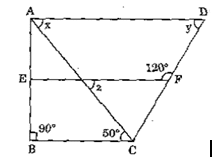• 30°
• 45°
• 60°
• 65°
3. In the figure given above ∠PQR=90°and QL is a median, PQ=5cm and QR=12cm,than QL is equal to ?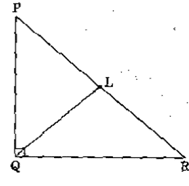• 5 cm
• 5 cm
• 6 cm
• 5 cm
1. Consider the following statements :
2. The tangent of a circle is a line that meets the circle in one and only one point
3. The tangent of a circle at the end point of the diameter is perpendicular to the diameter,

Which of the above statements is/are correct?

• 1 only
• 2 only’
• Both 1 and 2
• Neither 1 nor 2
1. In a right-angled triangle ABC, ∠C =90° and CD is perpendicular to AB, If AB x CD = CA x CB, then 1/CD2 is equal to

1/AB2-1/CA2

1/AB2-1/CB2

1/BC2+1/CA2

1/BC2-1/CA2 if CA>CB

1. The mean of 7 observations is 7. If each observation is increased by 2, then the new mean is
• 12
• 10
• 9
• 8
1. Which of the following are the examples of discrete variables?
2. Number of errors per page in. a book.
3. Height of individuals measured in cm.
4. Waiting time to failure of electric bulbs,
5. Number of leaves on branches of a tree.

Select the correct answer using the code given below :

• 1 only
• 1 and 4
• 3 and 4
• 2 and 4
1. The average weight of a class of 15 boys and 10 girls is 38.4 kg. If the average weight of the boy’s is 40 kg, then what is the average weight of the girls ?
• 5 kg
• 35 kg
• 36 kg
• 6 kg

Directions : Consider the following for the next three (03) questions that follow :

In a frequency distribution having class intervals 0 – 10, 10 – 20, 20 – 30 and 30 – 40, the respective frequencies are x, x + 8, x – 2 and x – 4 and the arithmetic mean of the distribution is 17.8.

1. The value of x is
• 3
• 6
• 8
• 12
1. The median lies in which one of the following, class intervals ?
• 0-10
• 10 – 20
• 20-30
• 30-40
2. The modal class is
• 0-10
• 10 – 20
• 20 – 30
• 30 – 40
3. Consider the following statements :
4. A frequency distribution condenses the data and reveals its important features.
5. A frequency distribution is an equivalent representation of original data.

which of the above statements is/are correct ?

• 1 only
• 2 only
• Both 1 and 2
• Neither 1 nor 2
1. Which one of the following statements is correct ?
• A frequency polygon is obtained by connecting the comer points of the rectangles in a histogram.
• A frequency polygon is, obtained by connecting the mid-points of the tops of the rectangles in a histogram.
• A frequency polygon is obtained by connecting the corner points of the class intervals in a histogram.
• None of the above